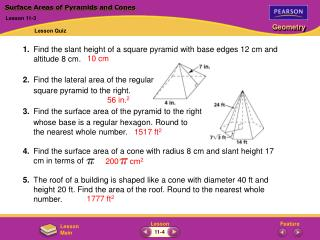# Surface Areas of Pyramids and Cones - PowerPoint PPT PresentationDownload PresentationSurface Areas of Pyramids and Cones

Surface Areas of Pyramids and ConesDownload Presentation## Surface Areas of Pyramids and Cones

- - - - - - - - - - - - - - - - - - - - - - - - - - - E N D - - - - - - - - - - - - - - - - - - - - - - - - - - -
##### Presentation Transcript

1. 1. Find the slant height of a square pyramid with base edges 12 cm and altitude 8 cm. 2. Find the lateral area of the regular square pyramid to the right. 3. Find the surface area of the pyramid to the right whose base is a regular hexagon. Round to the nearest whole number. 4. Find the surface area of a cone with radius 8 cm and slant height 17 cm in terms of . 5. The roof of a building is shaped like a cone with diameter 40 ft and height 20 ft. Find the area of the roof. Round to the nearest whole number. 200 cm2 Surface Areas of Pyramids and Cones Lesson 11-3 Lesson Quiz 10 cm 56 in.2 1517 ft2 1777 ft2 11-4

2. A = ½ bh = ½ (8)(4 3) = 16 3 ≈ 27.7 in.2 Check Skills You’ll Need Volumes of Prisms and Cylinders Lesson 11-4 Check Skills You’ll Need (For help, go to Lessons 1-9 and 10-1.) Find the area of each figure. Round to the nearest tenth if necessary. 1. square - side length 7 cm 3. circle - radius 10 mm 5. rectangle - 14 in. by 11 in. 7. equilateral triangle - side length 8 in. 2. circle - diameter 15 in. 4. rectangle - 3 ft by 1 ft 6. triangle - base 11 cm, height 5 cm A = r2 = (7.5)2 = 56.25 ≈ 176.7 in.2 A = s2 = 72 = 49 cm2 A = lw = (3)(1) = 3 ft2 A = r2 = (10)2 = 100 ≈ 314.2 mm2 A = lw = (14)(11) = 154 in.2 A = ½ bh = ½ (11)(5) = 27.5 cm2 11-4

3. Volumes of Prisms and Cylinders Lesson 11-4 Notes The volumeof a three-dimensional figure is the number of nonoverlapping unit cubes of a given size that will exactly fill the interior. Cavalieri’s principle says that if two three-dimensional figures have the same height and have the same cross-sectional area at every level, they have the same volume. The area of each shaded cross section below is 6 cm2. Since the prisms have the same height, their volumes must be the same. 11-4

4. Volumes of Prisms and Cylinders Lesson 11-4 Notes 11-4

5. The area of the base B=w= 3  5 = 15. Volumes of Prisms and Cylinders Lesson 11-4 Additional Examples Finding Volume of a Rectangular Prism Find the volume of the prism below. V= Bh Use the formula for volume. = 15 • 5Substitute 15 for B and 5 for h. = 75Simplify. The volume of the rectangular prism is 75 in.3. Quick Check 11-4

6. Use the Pythagorean Theorem to calculate the length of the other leg. 292 – 202 = 841  400 = 441  21 Volumes of Prisms and Cylinders Lesson 11-4 Additional Examples Finding Volume of a Triangular Prism Find the volume of the prism below. The prism is a right triangular prism with triangular bases. The base of the triangular prism is a right triangle where one leg is the base and the other leg is the altitude. 11-4

7. 1 2 1 2 The area B of the base is bh= (20)(21) = 210. Use the area of the base to find the volume of the prism. Volumes of Prisms and Cylinders Lesson 11-4 Additional Examples (continued) V= Bh Use the formula for the volume of a prism. = 210 •40Substitute. = 8400Simplify. The volume of the triangular prism is 8400 m3. Quick Check 11-4

8. The formula for the volume of a cylinder is V= r 2h. The diagram shows h and d, but you must find r. 1 2 r= d = 8 V= r 2h Use the formula for the volume of a cylinder. = • 82•9Substitute. = 576Simplify. The volume of the cylinder is 576 ft3. Volumes of Prisms and Cylinders Lesson 11-4 Additional Examples Finding Volume of a Cylinder Find the volume of the cylinder below. Leave your answer in terms of . Quick Check 11-4

9. You can use three rectangular prisms to find the volume. Volumes of Prisms and Cylinders Lesson 11-4 Additional Examples Finding Volume of a Composite Figure Find the volume of the composite space figure. Each prism’s volume can be found using the formula V = Bh. 11-4

10. Volumes of Prisms and Cylinders Lesson 11-4 Additional Examples (continued) Volume of prism I = Bh = (14 • 4) • 25 = 1400 Volume of prism II = Bh = (6 • 4) • 25 = 600 Volume of prism III = Bh = (6 • 4) • 25 = 600 Sum of the volumes = 1400 + 600 + 600 = 2600 The volume of the composite space figure is 2600 cm3. Quick Check 11-4

11. Volumes of Prisms and Cylinders Lesson 11-4 Lesson Quiz Find the volume of each figure to the nearest whole number. 1. 2. 3. 4.5. 62 m3 1800 ft3 45 in.3 63 m3 1800 mm3 11-4